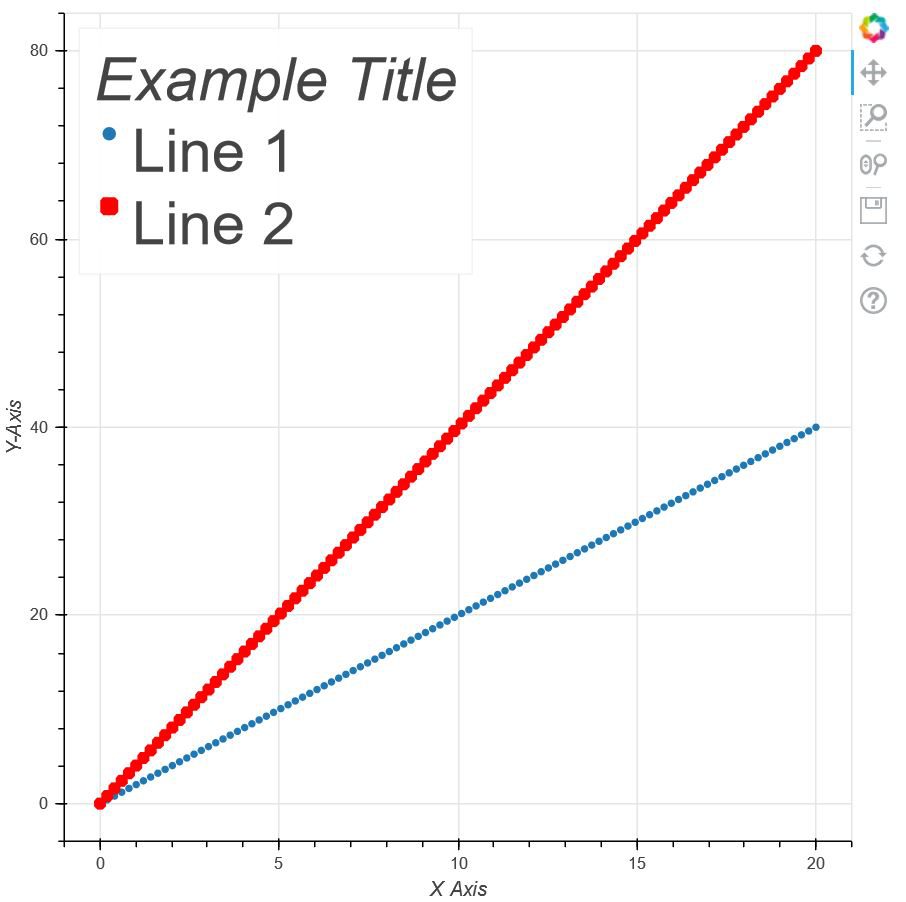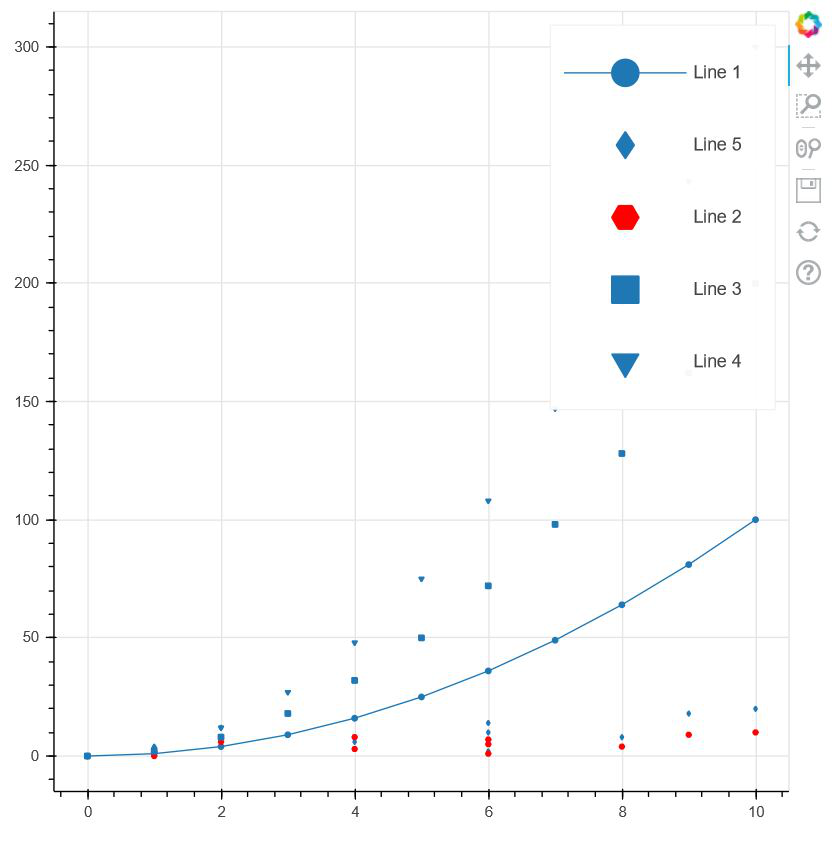Open In App

# How to change size of labels in the Bokeh legend?

In this article, we will be learning about how to change the size of labels in bokeh legend. Legend plays a very important role in bokeh. With the help of legend, we can point to various types of glyphs in a plot that bokeh provides us, and can customize them according to our choice. Along with that, we can also change various properties of the glyphs text shown in a box in a plot. Apart from that, there are various attributes that we can use to customize a legend.

Some of the attributes that can be used to customize a glyph: legend.label_text_font, legend.label_text_font_size, legend.location, legend.title, legend.orientation and, legend.clicking_policy.

Now, before proceeding further, we must be sure to have bokeh installed on our local device. If not, then open a command prompt and type the following command:

`pip install bokeh `

Now we are ready to go. It is necessary to install bokeh, else the functionalities won’t work. So, let us move to the implementation of the above concept.

Example 1:

In the following example, we will be changing the font size of the labels using bokeh legend and along with that, we will be learning some other features too.

## Python3

 `# importing numpy package fro python library``import` `numpy as np` `# import figure and show for creating``# and showing the plot from bokeh.plotting``from` `bokeh.plotting ``import` `figure,  show` `# Initialising a variable x with``# 100 values from 0 to 20``x ``=` `np.linspace(``0``, ``20``, ``100``)` `# initialising y with double values of``# x``y ``=` `x``*``2` `# Creating an empty figure with plot height and``# width as 600``p1 ``=` `figure(plot_width``=``600``, plot_height``=``600``)` `# Creating the plot with points``# in the shape of the circle``p1.circle(x, y, legend_label``=``"Line 1"``)` `# Creating the plot with points``# in the shape of the square``p1.square(x, ``2``*``y, legend_label``=``"Line 2"``,``          ``color``=``"red"``, line_width``=``4``)` `# using legend we are labelling the title of the``# Glyph box``p1.legend.title ``=` `'Example Title'` `# Labelling the X-Axis``p1.xaxis.axis_label ``=` `"X Axis"` `# Labelling the Y-Axis``p1.yaxis.axis_label ``=` `"Y-Axis"` `# Setting up the location of the``# box that differentiates the two``# lines``p1.legend.location ``=` `"top_left"` `# Increasing the title of the box``# to 30pt``p1.legend.title_text_font_size ``=` `'30pt'` `# Increasing the labels of the box``# to 30pt``p1.legend.label_text_font_size ``=` `"30pt"` `# Showing the above plot``show(p1)`

Output:Explanation:

In the above code, after initializing all the necessary packages from the libraries and modules, we are creating two points x and y which are a list of arbitrary values. Now we will be plotting those values in our graph. So, for that, we are creating an empty figure/plot whose plot width and height are given as 600. After that, we are plotting the two graphs where points on the first graph are in the shape of a circle and the points on the second graph are in the shape of a square. Apart from the different colors of the two graphs, in order to differentiate between them, we are creating a box where we are providing the labels for both the glyphs using legend_label and we are also giving a title to that box. Now comes the main implementation i.e changing the size of the labels using bokeh legend. So, by using legend.label_text_font_size we are customizing the size of the labels and glyphs in the box, and since we have given a title to the box, legend.label_text_font_size helps us to change the size of the title. Along with changing the size, we are also changing its orientation i.e on the top-left corner using legend.location.

Example 2:

Now, in the second example, lets us explore some more attributes of how to change sizes using legend. In the example below, instead of using label_text_font_size, we can also use glyph_height, glyph_width, label_height, label_width which are responsible for changing the labels and the glyph sizes separately in the label box differentiating all the lines. Let us move to the main implementation now.

## Python3

 `# importing numpy as np``import` `numpy as np` `# importing figure and show from``# bokeh.plotting``from` `bokeh.plotting ``import` `figure, show` `# creating a list of numbers from 0-10``x ``=` `np.arange(``11``)` `# Creating square of the numbers and``# storing it in y``y ``=` `x``*``*``2` `# Creating an array of random values in``# z``z ``=` `[``1``, ``6``, ``1``, ``4``, ``8``, ``6``, ``2``, ``6``, ``4``, ``9``, ``10``]` `# Creating a plot with plot width and height``# as 600``p ``=` `figure(plot_height``=``600``, plot_width``=``600``)` `# Plotting first line in the form of circle``p.circle(x, y, legend_label``=``"Line 1"``)` `# Creating first line in the form of line``p.line(x, y, legend_label``=``"Line 1"``)` `# Creating fifth line in the form of line``p.diamond(z, x``*``2``, legend_label``=``"Line 5"``)` `# Creating second line in the form of line``p.``hex``(z, x, legend_label``=``"Line 2"``, color``=``"red"``)` `# Creating third line in the form of line``p.square(x, y``*``2``, legend_label``=``"Line 3"``)` `# Creating forth line in the form of line``p.inverted_triangle(x, y``*``3``, legend_label``=``"Line 4"``)` `# Increasing the glyph height``p.legend.glyph_height ``=` `50` `# increasing the glyph width``p.legend.glyph_width ``=` `90` `# Increasing the glyph's label height``p.legend.label_height ``=` `50` `# Increasing the glyph's label height``p.legend.label_width ``=` `50` `# showing the above plot``show(p)`

Output:Explanation:

In the above code, we have imported numpy package along with the figure and show for plot creation and showing. After importing the packages, we are creating three different lists of numbers which are then plotted against each other in graphical format. They are of different shapes. Using legend in bokeh, we are labeling them differently, and using the following attributes mentioned above, we are changing the size of the glyphs and the labels separately which also affects the box size.